Examples

Practical Geometry
Serial order wise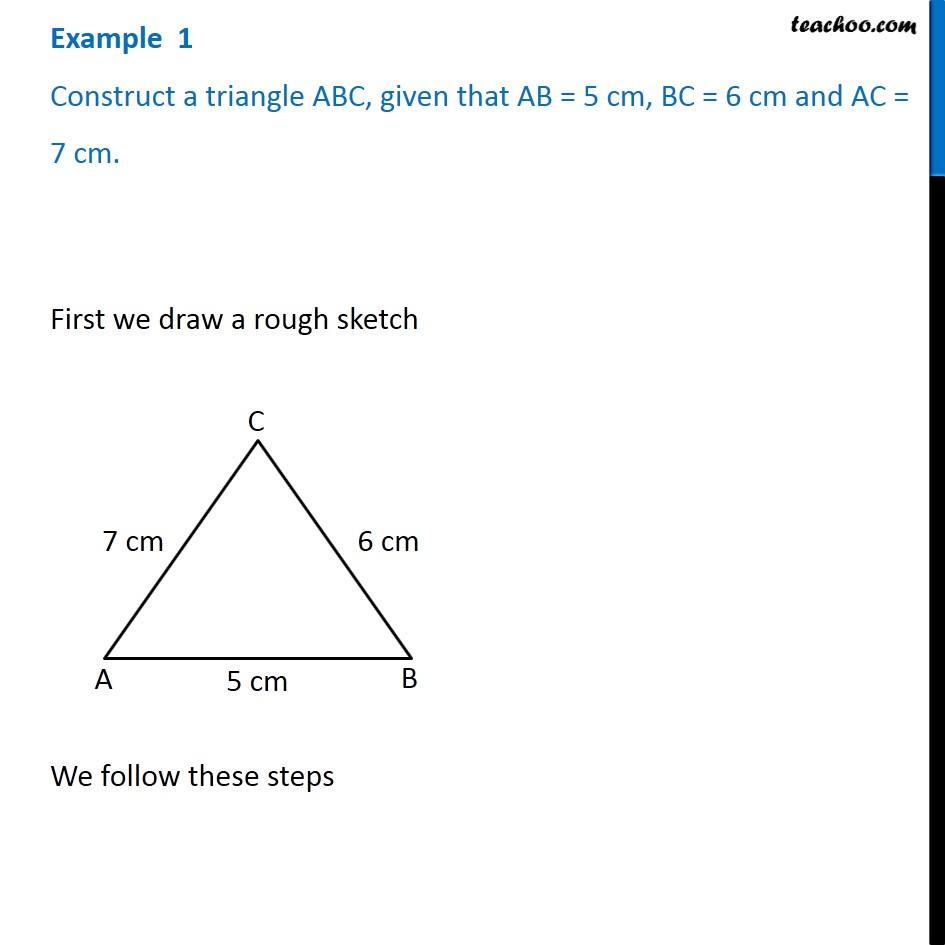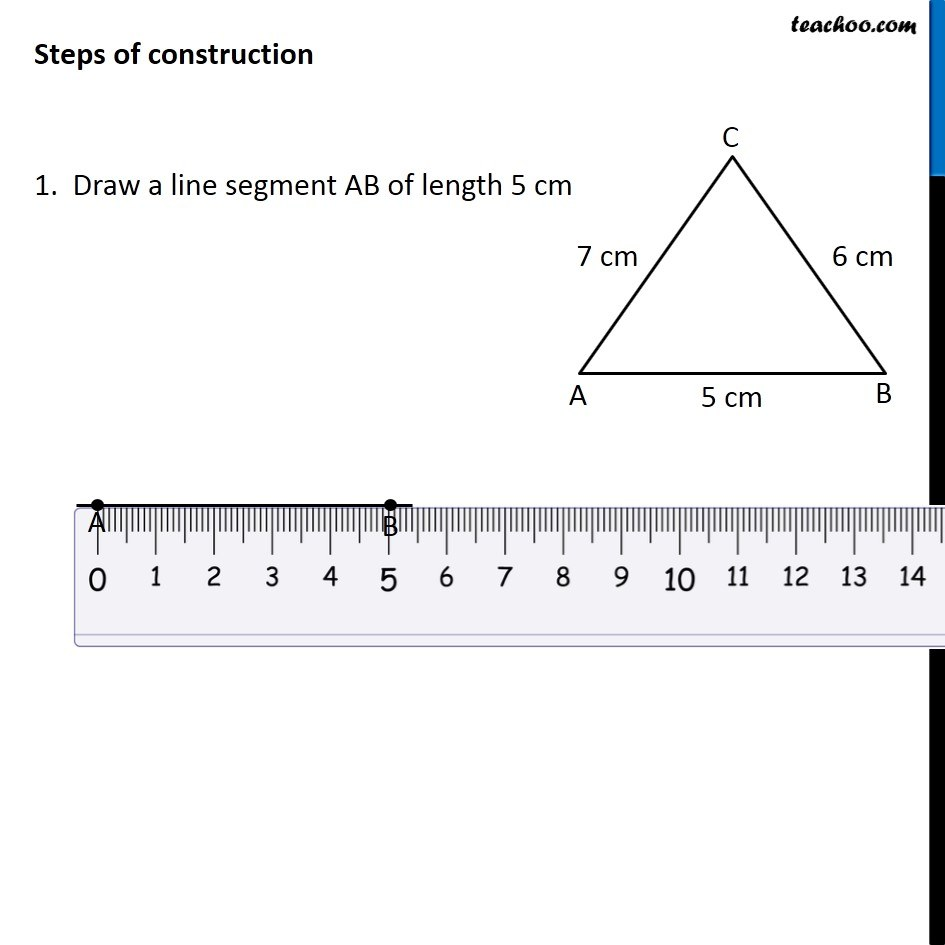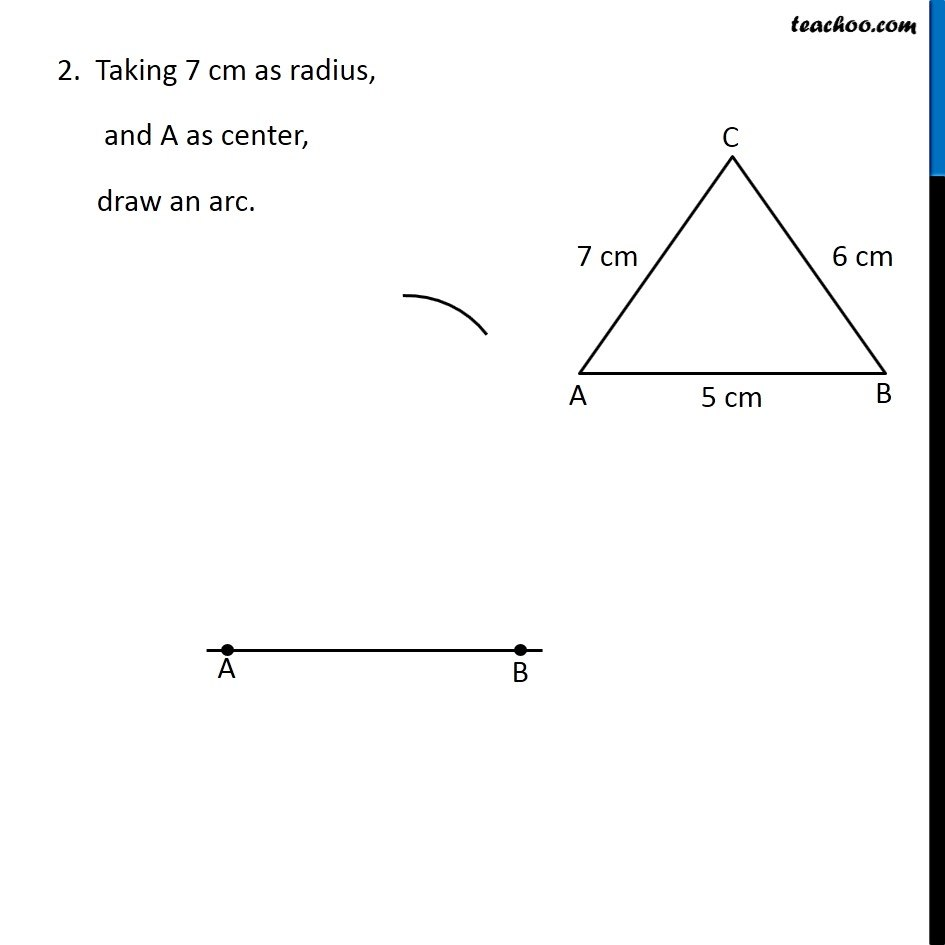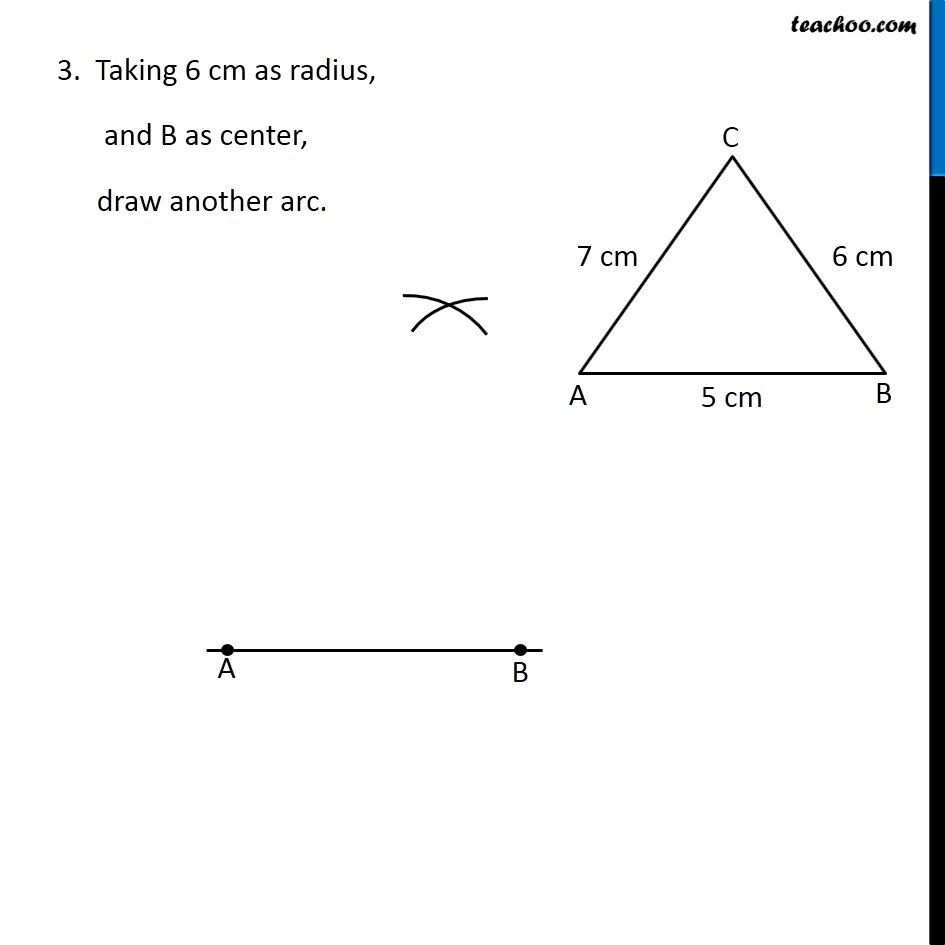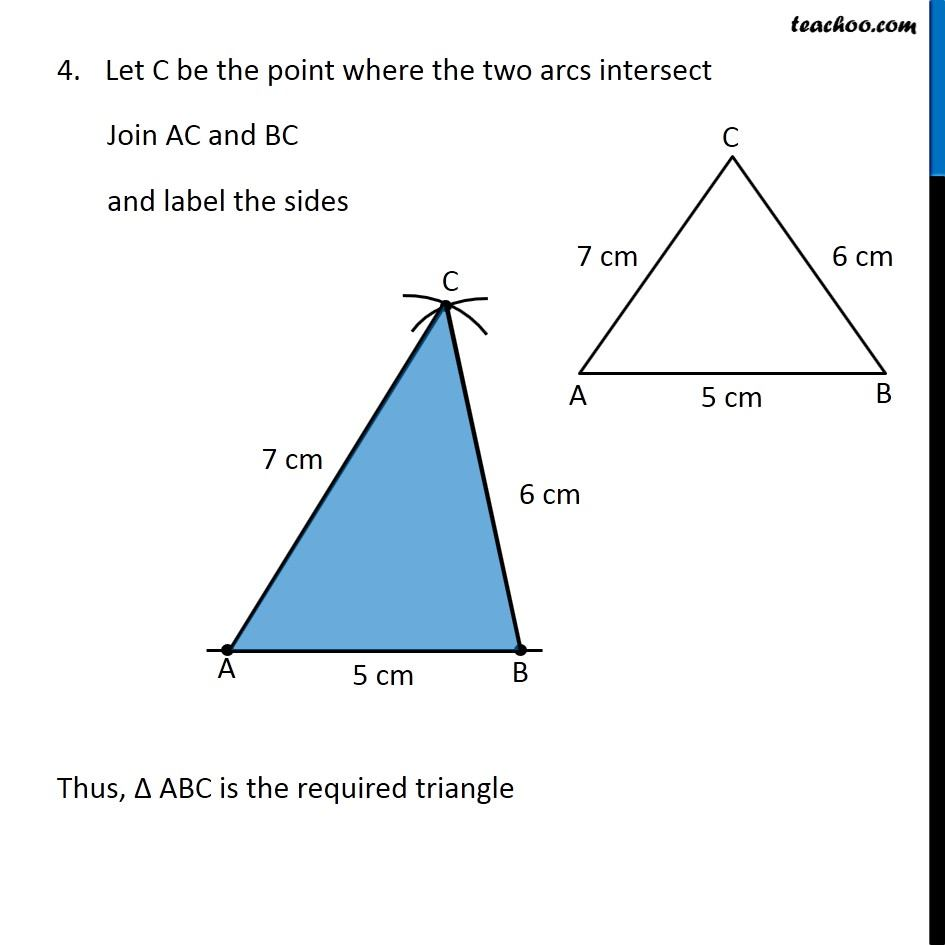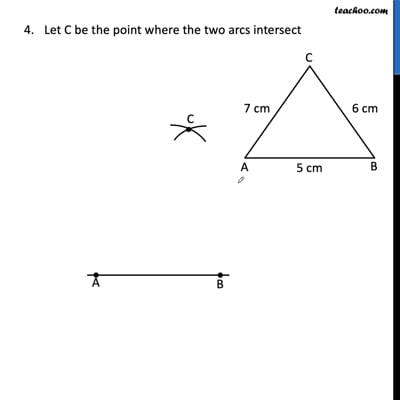This video is only available for Teachoo black users

Learn in your speed, with individual attention - Teachoo Maths 1-on-1 Class

### Transcript

Example 1 Construct a triangle ABC, given that AB = 5 cm, BC = 6 cm and AC = 7 cm. First we draw a rough sketch We follow these steps Steps of construction 1. Draw a line segment AB of length 5 cm 2. Taking 7 cm as radius, and A as center, draw an arc. 3. Taking 6 cm as radius, and B as center, draw another arc. Let C be the point where the two arcs intersect Join AC and BC and label the sides Thus, Δ ABC is the required triangle